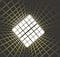# Implement Convolutional Layer in Python

## CNN Explained

You probably have used convolutional functions from Tensorflow, Pytorch, Keras, or other deep learning frameworks. But in this article, I would like to implement the convolutional layers from scratch, which, I believe, could help one gains a deeper understanding of each component in the convolutional process.Photo by Dhruv on Unsplash

We are going to implement the forward propagation with 4 different steps:

• Zero Paddings
• Convolutional Layer
• Pooling
• Conv + Pool

Let’s start with padding.

# Zero Paddings

Zero Padding pads 0s at the edge of an image, benefits include:
1. It allows you to use a CONV layer without necessarily shrinking the height and width of the volumes. This is important for building deeper networks since otherwise the height/width would shrink as you go to deeper layers. An important special case is the “same” convolution, in which the height/width is exactly preserved after one layer.

2. It helps us keep more of the information at the border of an image. Without padding, very few values at the next layer would be affected by pixels at the edges of an image.

Consider an input of batched images with shape:

Where `m` is the batch size, `n_W` is the width of the image, `n_H` is the height and `n_C` is the number of channels — RGB would have 3 channels.

After padded with size `p`, the size would become

# One Step of Convolutional Layer

Consider a filter mapped to one piece of the image, with

Where filter has the depth of the piece of the input image.

Another way to look at this is you can think of the filter as the weights `W`, and for each piece of the image, it serves as an input `X`, so in the convolutional process, the formula equals:

Where `b` is the bias and `g` is the activation function. Doesn’t it look very similar to the equations in the dense neural network?

# Convolution

Now the input (here we use `A_prev` ) would be a batch of whole images with size

Filter with size

Where `n_C` is the number of filters, which would become the depth of the output image.

Bias with size

And parameters include:

So the resulting output would have size:

Now given an image from the input, we will need to slice it into pieces and multiply with the filter one by one.

Consider a 2D image with size `n_W_prev, n_H_prev`, and stride is `s`, filter size of `f`, then the top-left corner of the output image would have mapping:

We will make use of this pattern in our implementation of slice the original image and map to the output.

# Pooling

After the convolutional layer, it typically follows a pooling layer. The pooling (POOL) layer reduces the height and width of the input. It helps reduce computation, as well as helps make feature detectors more invariant to its position in the input. The two types of pooling layers are:

- Max-pooling layer: slides an `(f, f)` window over the input and stores the max value of the window in the output.

- Average-pooling layer: slides an `(f, f)` window over the input and stores the average value of the window in the output.

The process is pretty much the same as the convolutional layer, with a filter and a stride, at each step, we will take a slice of the whole image and compute one value — either max or average — from it.

Given filter size `f`, stride `s` and input size:

The output would have size:

Note that pooling does not change the depth of an image.

Hence, we’ve finished the forward propagation of a convolutional layer, for the backward propagation you can check the explanation here.

For a more formatted and completed code guide, please refer to my Github.

This article originates from the deep learning specialization course.

Hmm…I am a data scientist looking to catch up the tide…

## More from Jeremy Zhang

Hmm…I am a data scientist looking to catch up the tide…